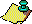Contents Next: How many elements Up: The 3x+1 problem. Previous: Behavior of the

Theorem C shows that generally they are equal: For any fixed k at most a finite number of those n having coefficient stopping time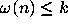have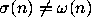. Terras  and later Garner  conjecture that this never occurs.

### COEFFICIENT STOPPING TIME CONJECTURE.

For all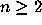, the stopping time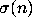equals the coefficient stopping time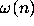.

The Coefficient Stopping Time Conjecture has the aesthetic appeal that if it is true, then the set of positive integers with stopping time k is exactly a collection of congruence classes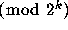, as described by part (i) of Theorem C. Furthermore, the truth of the Coefficient Stopping Time Conjecture implies that there are no nontrivial cycles. To see this, suppose that there were a nontrivial cycle of period k and let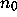be its smallest element, and note that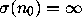. Then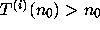for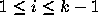and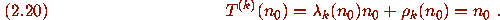Now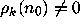sinceisn't a power of 2, so that (2.20) implies that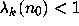. Hence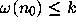, so that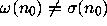. The following result shows that the Coefficient Stopping Time Conjecture is ``nearly true.'' I will use it later to bound the number of elements not having a finite stopping time.

### Theorem E

There is an effectively computable constant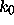such that if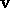is admissible of length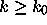, then all elements of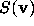have stopping time k except possibly the smallest element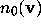of S.

### [Proof]Contents Next: How many elements Up: The 3x+1 problem. Previous: Behavior of the